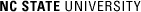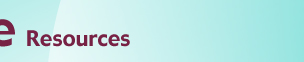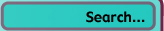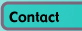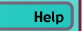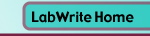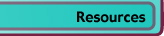# Graphing Resources

## Line Graphs and Scatter Plots

• One Independent and One Dependent Variable
• Two (or More) Independent Variables and One Dependent Variable
• Excel Tips

### Introduction

Line graphs provide an excellent way to map independent and dependent variables that are both quantitative. When both variables are quantitative, the line segment that connects two points on the graph expresses a slope, which can be interpreted visually relative to the slope of other lines or expressed as a precise mathematical formula. Scatter plots are similar to line graphs in that they start with mapping quantitative data points. The difference is that with a scatter plot, the decision is made that the individual points should not be connected directly together with a line but, instead express a trend. This trend can be seen directly through the distribution of points or with the addition of a regression line. A statistical tool used to mathematically express a trend in the data.

1. ### Scatter Plot

2.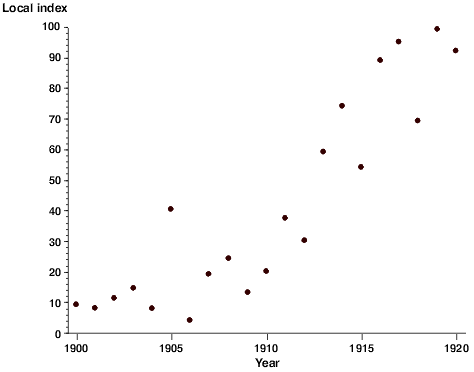With a scatter plot a mark, usually a dot or small circle, represents a single data point. With one mark (point) for every data point a visual distribution of the data can be seen. Depending on how tightly the points cluster together, you may be able to discern a clear trend in the data.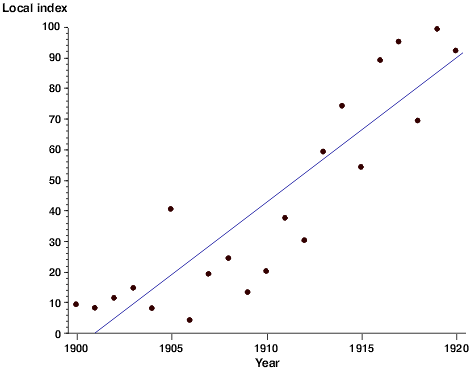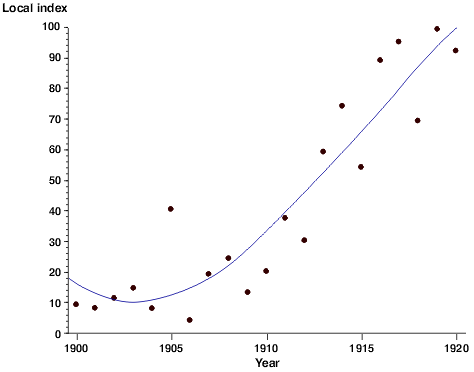Because the data points represent real data collected in a laboratory setting rather than theoretically calculated values, they will represent all of the error inherent in such a collection process. A regression line can be used to statistically describe the trend of the points in the scatter plot to help tie the data back to a theoretical ideal. This regression line expresses a mathematical relationship between the independent and dependent variable. Depending on the software used to generate the regression line, you may also be given a constant that expresses the 'goodness of fit' of the curve. That is to say, to what degree of certainty can we say this line truly describes the trend in the data. The correlational constant is usually expressed as R2 (R-squared). Whether this regression line should be linear or curved depends on what your hypothesis predicts the relationship is. When a curved line is used, it is typically expressed as either a second order (cubic) or third order (quadratic) curve. Higher order curves may follow the actual data points more closely, but rarely provide a better mathematical description of the relationship.

3. ### Line Graph

4.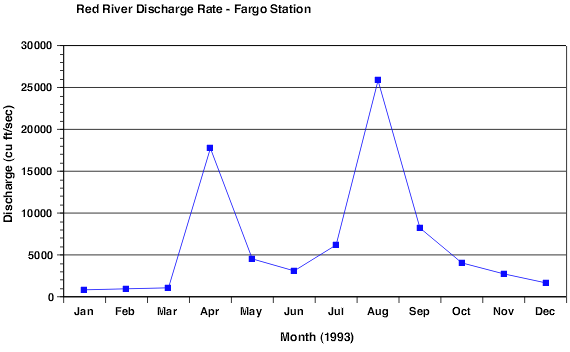Line graphs are like scatter plots in that they record individual data values as marks on the graph. The difference is that a line is created connecting each data point together. In this way, the local change from point to point can be seen. This is done when it is important to be able to see the local change between any to pairs of points. An overall trend can still be seen, but this trend is joined by the local trend between individual or small groups of points. Unlike scatter plots, the independent variable can be either scalar or ordinal. In the example above, Month could be thought of as either scalar or ordinal. The slope of the line segments are of interest, but we would probably not be generating mathematical formulas for individual segments.

The above example could have also been produced as a bar graph. You would use a line graph when you want to be able to more clearly see the rate of change (slope) between individual data points. If the independent variable was nominal, you would almost certainly use a bar graph instead of a line graph.

1. ### Multiple Line Graph

2.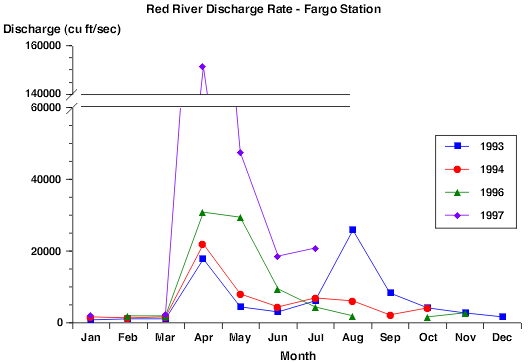Here, we have taken the same graph seen above and added a second independent variable, year. Both the independent variables, month and year, can be treated as being either as ordinal or scalar. This is often the case with larger units of time, such as weeks, months, and years. Since we have a second independent variable, some sort of coding is needed to indicate which level (year) each line is. Though we could label each bar with text indicating the year, it is more efficient to use color and/or a different symbol on the data points. We will need a legend to explain the coding scheme.

Multiple line graphs have space-saving characteristics over a comparable grouped bar graph. Because the data values are marked by small marks (points) and not bars, they do not have to be offset from each other (only when data values are very dense does this become a problem). Another advantage is that the lines can easily dual coded. With the lines, they can both be color coded (for computer and color print display) or shape coded with symbols (for black & white reproduction). With bars, shape coding cannot be used, and pattern coding has to be substituted. Pattern coding tends to be much more limiting.

Notice that there is a break in the 1996 data line (green/triangle) between August and October. Because the data point for September is missing, the line should not be connected between August and October since this would give an erroneous local slope. This is particularly important if you display the line without symbols at individual data points.

### Excel Tips

For information on creating bar graphs with Excel, go to the Scatter Plots and Line Graphs Module, or go to the Excel Tutorial Main Menu for a complete list of modules.

### Specific tips for line graphs

• The graphing tutorial gives specific instructions on creating scatter plots and regression lines
• Line graphs can be created with either the Line Graph type or with (XY) Scatter. When using (XY) Scatter, choose the Connected with Line sub-type.
• It is simpler to create a line graph with (XY) Scatter when your independent and dependent variables are in columns.
• Marks for data points are called Markers
• The color and size of the line and markers can be set by double-clicking on the line in the graph.
• Markers can be turned off by double-clicking the line and choosing None under Markers.© Copyright NC State University 2004 Sponsored and funded by National Science Foundation (DUE-9950405 and DUE-0231086) Site design by Rosa Wallace Rev. RW 5/16/05